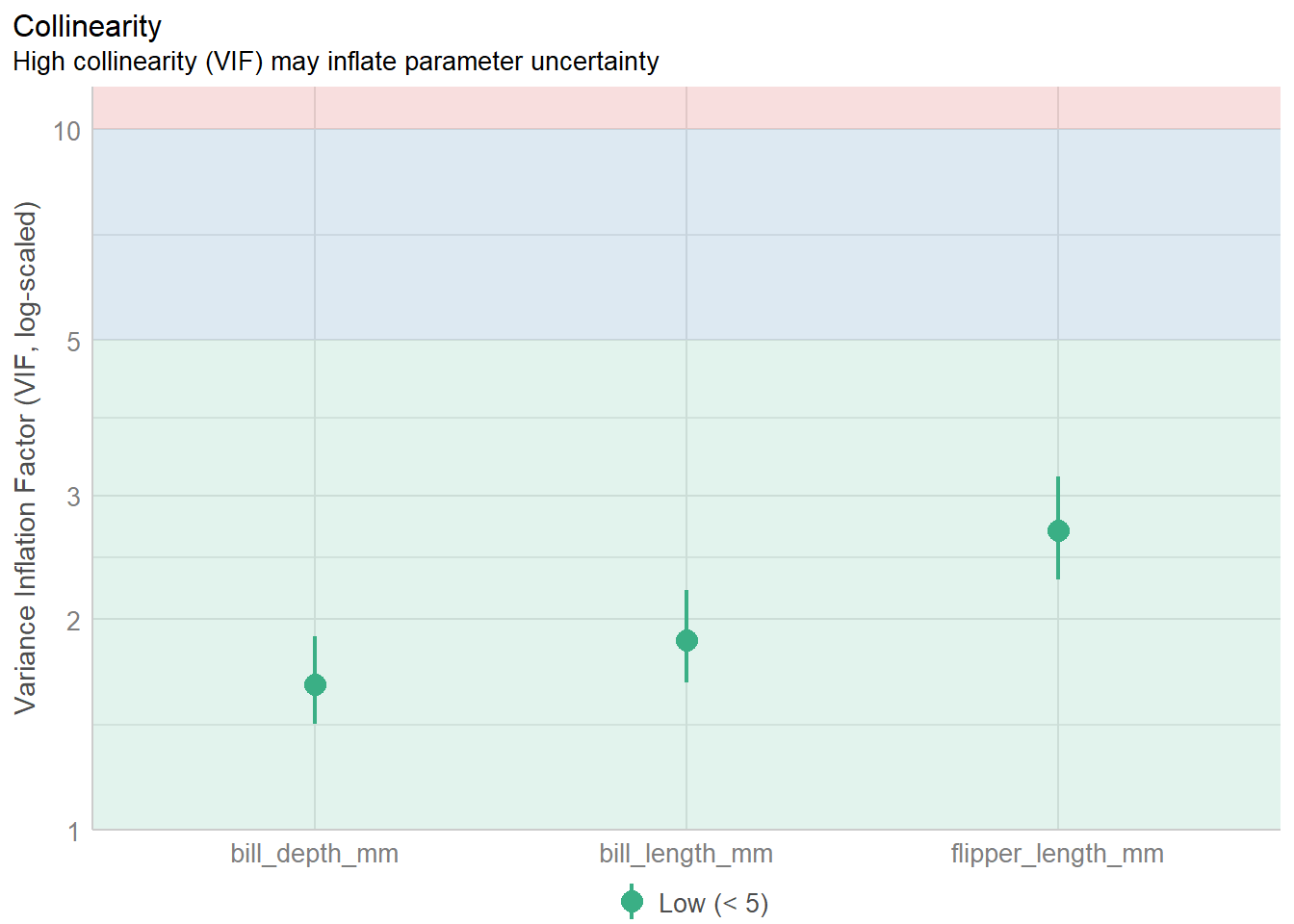## 10.3 Multicollinearity

• Occurs when some of the X variables are highly intercorrelated.
• Computed estimates of regression coefficients are unstable and have large standard errors.

For example, the squared standard error of the $$i$$th slope coefficient ($$[SE(\beta_{i})]^2$$) can be written as:

$[SE(\beta_{i})]^2 = \frac{S^{2}}{(N-1)(S_{i}^{2})}*\frac{1}{1 - (R_{i})^2}$

where $$S^{2}$$ is the residual mean square, $$S_{i}$$ the standard deviation of $$X_{i}$$, and $$R_{i}$$ the multiple correlation between $$X_{i}$$ and all other $$X$$’s.

When $$R_{i}$$ is close to 1 (very large), $$1 - (R_{i})^2$$ becomes close to 0, which makes $$\frac{1}{1 - (R_{i})^2}$$ very large.

This fraction is called the variance inflation factor and is available in most model diagnostics.

big.pen.model <- lm(body_mass_g ~ bill_length_mm + bill_depth_mm + flipper_length_mm, data=pen)
performance::check_collinearity(big.pen.model) |> plot()• Solution: use variable selection to delete some X variables.
• Alternatively, use dimension reduction techniques such as Principal Components (Chapter 14).